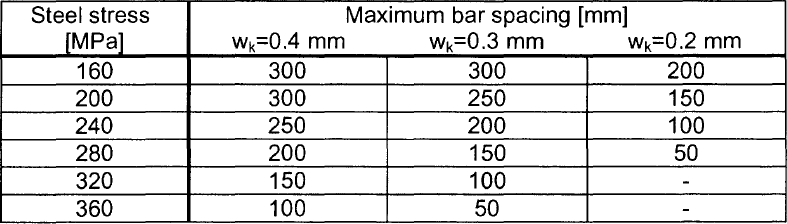# RF-CONCRETE Members – Online Manual Version 5

Online manuals, introductory examples, tutorials, and other documentation.

# 2.2.4 Crack Width Control

### Crack Width Control

Checking rebar diameter

The limit diameter of reinforcing bars with max Øs is checked in accordance with EN 1992-1-1, clause 7.3.3 (2) as follows.

where

• Øs* :  limit diameter according to Figure 2.3
• fct, eff : effective tensile strength of concrete at relevant point of time, in this case fctm
• kc : factor for considering stress distribution in tension zone, see chapter 2.2.3
• hcr :  depth of tension zone immediately before cracking occurs
• h : overall depth of cross-section
• d : effective depth up to the centroid of outside reinforcement
Design of rebar spacing

The maximum rebar spacing max sl is specified according to EN 1992-1-1, Table 7.3 (see Figure 2.4).Figure 2.4 Maximum values for rebar spacings according to EN 1992-1-1, Table 7.3
Design of crack width by direct calculation

The characteristic crack width wk is determined according to EN 1992-1-1, clause 7.3.4, Eq. (7.8).

Equation 2.12 EN 1992-1-1, Eq. (7.8)

where

 sr,max maximum crack spacing for final crack state according to Eq. (7.11) or (7.14) εsm mean strain of reinforcement considering contribution of concrete to tension between the cracks εcm mean strain of concrete between the cracks
Maximum crack spacing sr,max

If the rebar spacing in the tension zone is not greater than 5 ⋅ (c + Ø/2), the maximum crack spacing for the final crack state may be determined as follows according to EN 1992-1-1, clause 7.3.4 (3):

Equation 2.13 EN 1992-1-1, Eq. (7.11)

where

 k3 recommended value: 3.4 (German National Annex: 0) c concrete cover of longitudinal reinforcement k1 coefficient for considering the bond properties of the reinforcement (0.8 for ribbed steel bars and 1.6 for rebars with a plain surface) k2 coefficient for considering strain distribution (0.5 for bending and 1.0 for pure tension) k4 recommended value: 0.425 (German National Annex: 1/3.6) ρp,eff effective reinforcement ratio

If the spacing of rebars within the bond exceeds 5 ⋅ (c + Ø/2) or if there is no reinforcement within the bond in the tension zone, the following limit value of the crack width may be assumed:

Equation 2.14 EN 1992-1-1, Eq. (7.14)

Applying equations (7.11) and (7.14) are "optional" rules within the meaning of the Eurocode. Internal study of these two crack spacing equations has shown that the explicit differentiation when applying equation (7.14) to rebars with a larger spacing than 5 ⋅ (c + Ø/2) does not always lead to the desired crack width. We analyzed cross-sections with slightly different rebar spacings in the range of 5 ⋅ (c + Ø/2). For T-beam-like cross-sections and a bar spacing of 1.01 ⋅ [5 ⋅ (c + Ø/2)] using Eq. (7.14), the result was a smaller crack spacing than with Eq. (7.11) and a bar spacing of 0.99 ⋅ [5 ⋅ (c + Ø/2)]. This would mean that when you increase the reinforcement content, the crack width increases as soon as you fall below the limit value of the rebar spacing 5 ⋅ (c + Ø/2). To put it clearly: The calculated crack width in a zone without reinforcement is smaller than in a reinforced zone!

In the program, the crack spacing is calculated using equation (7.11) by default. Optionally, it is possible to activate sr,max as the upper limit according to equation (7.14). As a result of the circumstance described above, the upper limit value is always taken into account, regardless of the available rebar spacing in the tension reinforcement.

Difference of mean strain (εsm - εcm)

The difference of the mean strain of concrete and reinforcing steel is determined as follows according to  7.3.4 (2), Eq. (7.9).

Equation 2.15 EN 1992-1-1, Eq. (7.9)

where

• σs : stress in tension reinforcement assuming a cracked cross-section
• kt : factor for creep of bond
• kt = 0.6 for short-term loading
• kt = 0.4 for long-term loading
• fct,eff : effective tensile strength of concrete at relevant point of time (in this case fctm)
• αe : ratio of moduli of elasticity Es / Ecm
• ρeff : effective reinforcement ratio
Literatur
  Eurocode 2: Design of concrete structures - Part 1-1: General rules and rules for buildings; EN 1992-1-1:2011-01